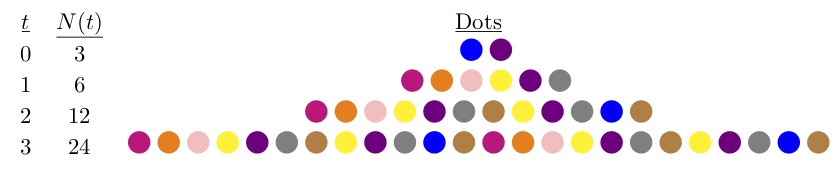# Exponential Growth

## Problem

On her computer, Alex has a screen saver that models exponential growth. The screen saver shows colored dots whole number can be modeled but the equation  where t stands for the number of seconds since the screen saver’s cycle started. One second after the cycle’s start, there were 6 dots on the screen; three seconds after the cycle’s start there were 24 dots. Find the value of a.

## Solution

What do you know? You’re told the equation is . And you know two data points for that equation:  and  What can you do with that?

Plug the known data points into the equation:That’s a system of two equations with two unknowns. You can solve it by substitution. Note that if you multiply the N(1) equation by 4, you get 24, the same value that equals N(3).

Replace the 24 in N(3) with

Divide by :

Exponential bases are always positive, so . And that’s the answer.To check, go back to one of the original equations and solve for  then plug both a and  into the other original equation and see if you get a true statement:

and

Check.